@coder-pig 2022-09-06T09:25:45.000000Z 字数 1350 阅读 172

# 小猪的C语言快速入门系列(七)

C语言

指针数组二级指针内存的动态 以及 const修饰指针

## 本节学习路线图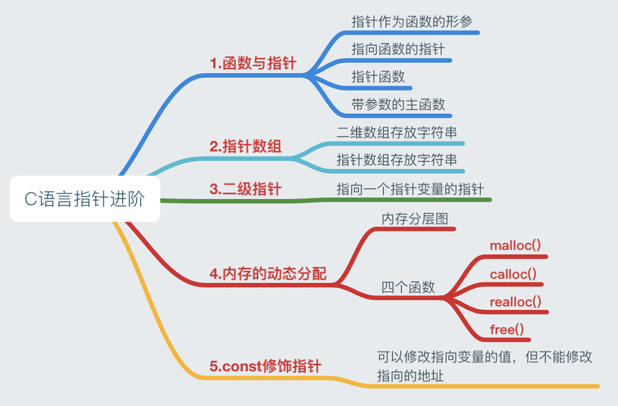## 1.函数与指针

### 1）指针作为函数的形参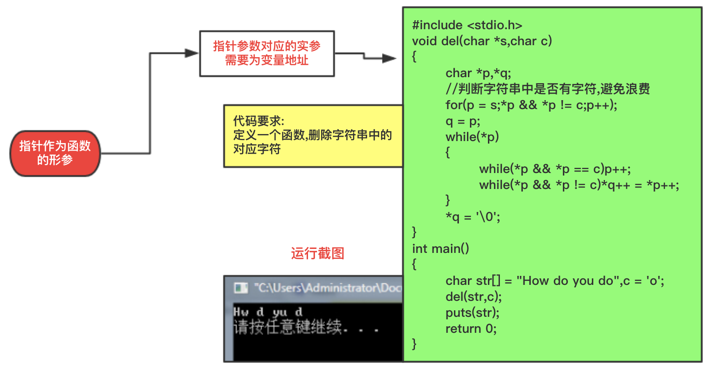### 2）指向函数的指针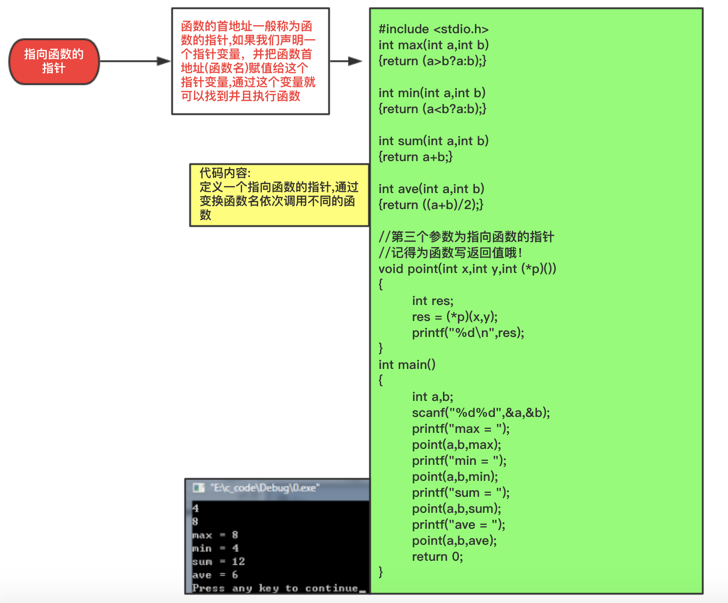### 3）指针函数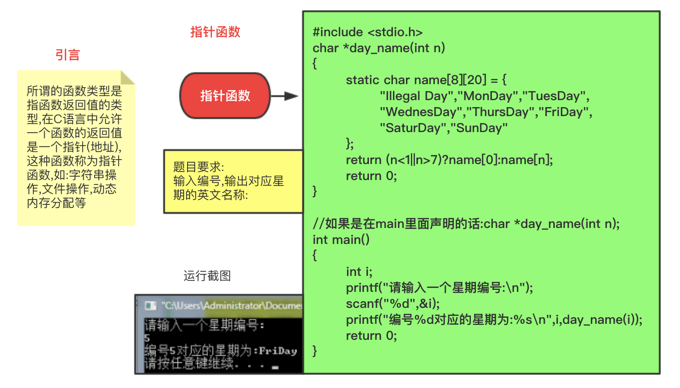### 4）带参数的主函数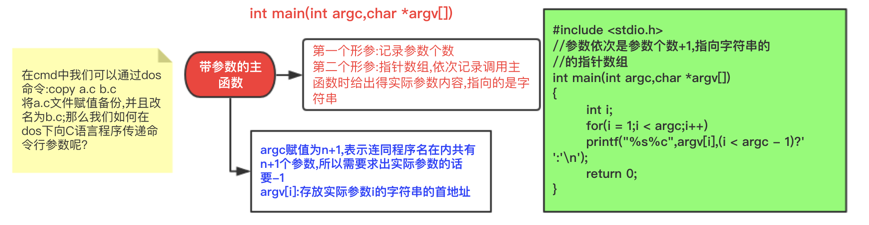## 2.指针数组

char name = "~" ②char *name = "~" //前者定义了长度,不能直接赋值,明显后者比较灵活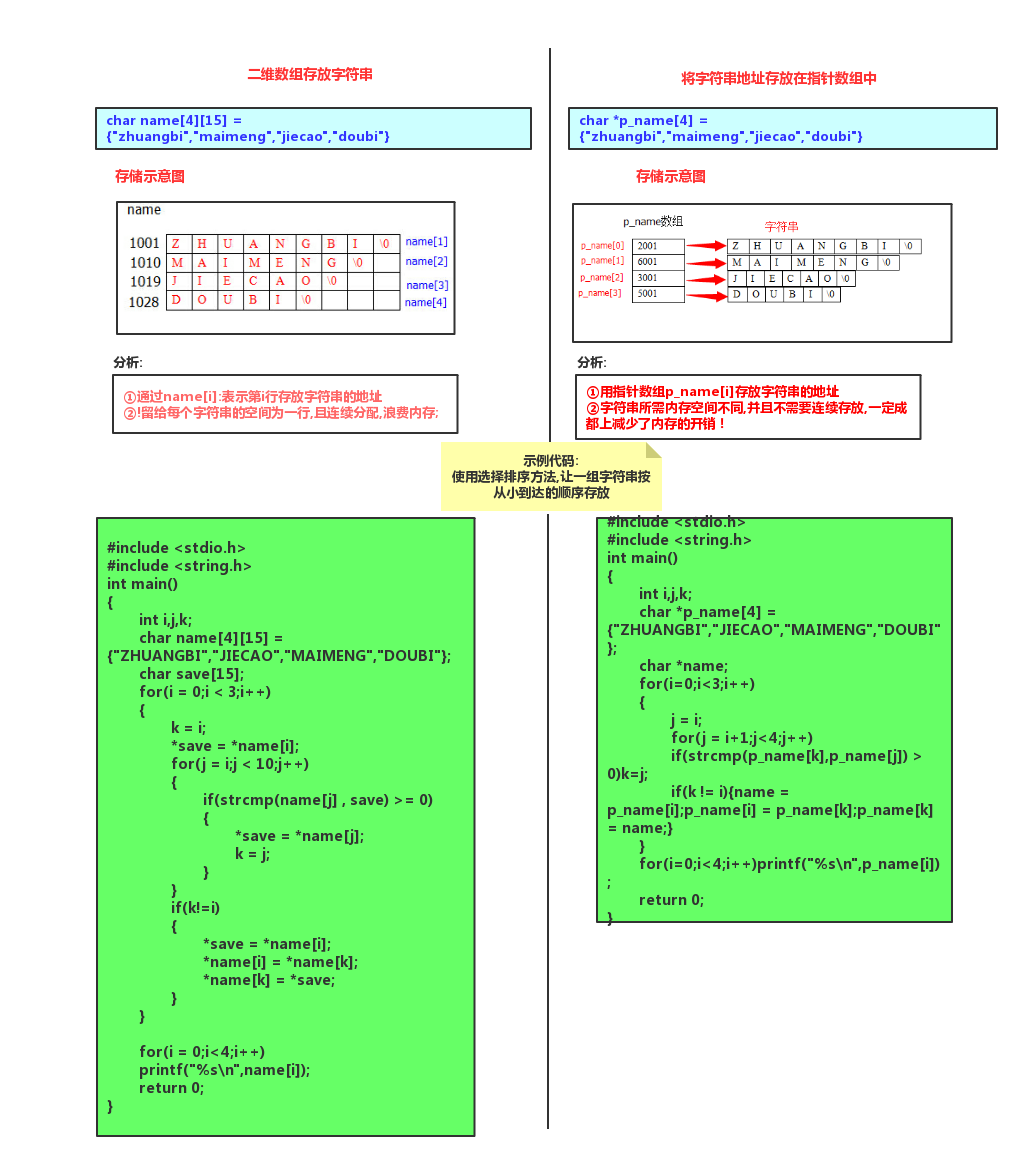## 3.二级指针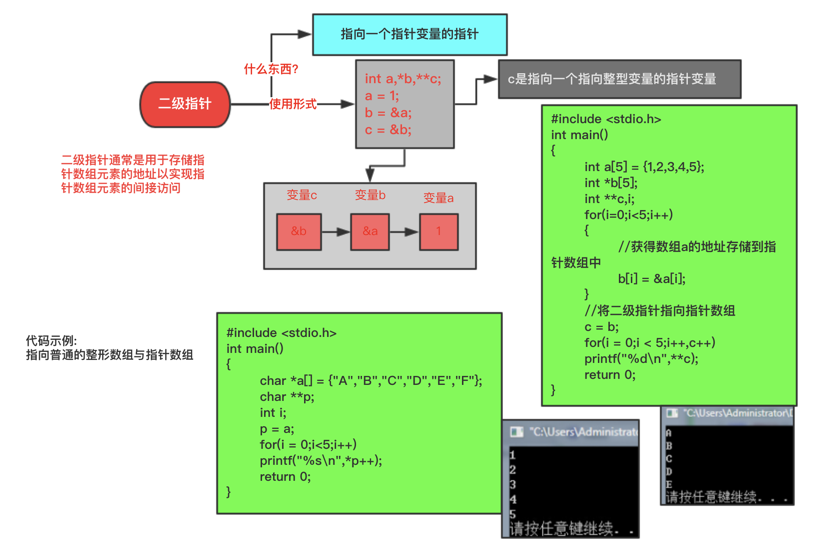## 4.内存的动态分配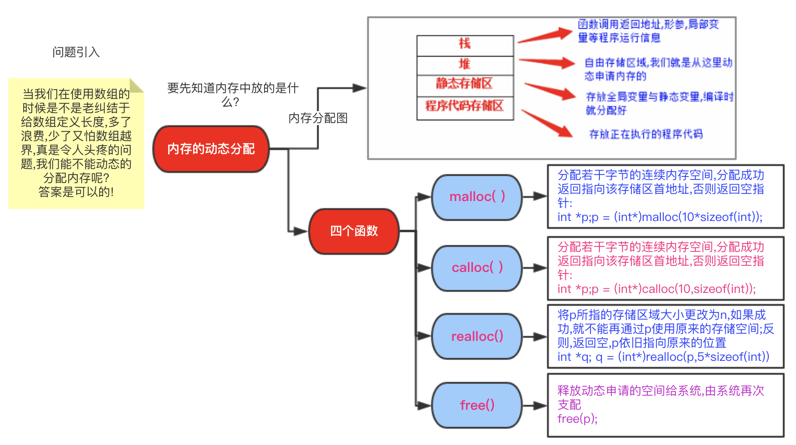#include <stdio.h>  #include <stdlib.h>  #define N 10  int main()  {      int *p = 0;      int  i,num,*q = 0;      p = (int *)malloc((N * sizeof(int)));      if(p == 0)      {          printf("内存分配错误!\n");          exit(0);      }      printf("请输入要存储的元素个数:\n");      scanf("%d",&num);      if(num <= N)      {          for(i = 0;i < N;i++)          scanf("%d",p+i);      }      else      {          for(i = 0;i < num;i++)          q = (int *)realloc(p,(N + N)*sizeof(int));          if(q == 0)exit(0);          for(i = 0;i < num;i++)          scanf("%d",q + i);          p = q;      }      for(i = 0;i < num;i++)      printf("%3d",*(p+i));      printf("\n");      free(p);      return 0;     }

## 5.使用const修饰指针变量

const 关键字修饰的数据类型是指 常类型，最简单的用法是用来定义const

const修饰的常量在声明的时候就要进行初始化赋值，不然后面是不能赋值的！

• int const *a; //修饰指向的内容，a可变，a指向的内容不可变
• int *const a; //修饰指针a，a不可变，a指向的内容可变
• const int *a; //修饰指向的内容，a可变，a指向的内容不可变
• const int *const A; //指针a和a指向的内容都不可变

#include<stdio.h>int main(){    int const a;    a = 5;    printf("a = %d\n",a);    return 0;}• 私有
• 公开
• 删除